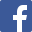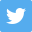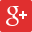THE HISTORY OF WIMBLEDON FOOTBALL CLUB
1923
Senior Section P W D L F A GD GA Pt Adj Reserve Section Isthmian League `1.` `St. Albans City` `26` `17` `5` `4` `72` `38` `34` `1.89` `39` `2.` `Dulwich Hamlet` `26` `15` `6` `5` `49` `28` `21` `1.75` `36` `3.` `Clapton` `26` `14` `5` `7` `73` `50` `23` `1.46` `33` `4.` `Wycombe Wanderers` `26` `14` `5` `7` `88` `65` `23` `1.35` `33` `5.` `London Caledonians` `26` `14` `3` `9` `53` `49` `4` `1.08` `31` `6.` `Civil Service` `26` `12` `5` `9` `52` `47` `5` `1.11` `29` `7.` `The Casuals` `26` `13` `1` `12` `65` `54` `11` `1.20` `27` `8.` `Ilford` `26` `9` `6` `11` `56` `59` `-3` `0.95` `24` `9.` `Nunhead` `26` `8` `8` `10` `41` `46` `-5` `0.89` `24` `10.` `Wimbledon` `26` `8` `4` `14` `43` `62` `-19` `0.69` `20` `11.` `Tufnell Park` `26` `8` `2` `16` `38` `53` `-15` `0.72` `18` `12.` `Woking` `26` `5` `8` `13` `31` `62` `-31` `0.50` `18` `13.` `Oxford City` `26` `7` `2` `17` `53` `74` `-21` `0.72` `16` `14.` `Leytonstone` `26` `6` `4` `16` `41` `68` `-27` `0.60` `16` ` ` `364` `150` `64` `150` `755` `755` `0` `364` `0` Isthmian League `1.` `Wimbledon (reserves)` `26` `18` `7` `1` `72` `25` `47` `2.88` `43` `2.` `Tufnell Park (reserves)` `26` `17` `5` `4` `66` `33` `33` `2.00` `39` `3.` `St. Albans City (reserves)` `26` `16` `5` `5` `91` `40` `51` `2.27` `37` `4.` `Dulwich Hamlet (reserves)` `26` `16` `5` `5` `83` `37` `46` `2.24` `37` `5.` `Clapton (reserves)` `26` `11` `6` `9` `50` `48` `2` `1.04` `28` `6.` `The Casuals (reserves)` `26` `10` `6` `10` `44` `38` `6` `1.16` `26` `7.` `Wycombe Wanderers (reserves)` `26` `11` `4` `11` `59` `67` `-8` `0.88` `26` `8.` `Woking (reserves)` `26` `10` `5` `11` `48` `57` `-9` `0.84` `25` `9.` `Ilford (reserves)` `26` `10` `3` `13` `48` `58` `-10` `0.83` `23` `10.` `London Caledonians (reserves)` `26` `8` `4` `14` `51` `60` `-9` `0.85` `20` `11.` `Civil Service (reserves)` `26` `9` `2` `15` `45` `69` `-24` `0.65` `20` `12.` `Nunhead (reserves)` `26` `9` `1` `16` `45` `69` `-24` `0.65` `19` `13.` `Leytonstone (reserves)` `26` `5` `4` `17` `42` `71` `-29` `0.59` `14` `14.` `Oxford City (reserves)` `26` `2` `3` `21` `32` `104` `-72` `0.31` `7` ` ` `364` `152` `60` `152` `776` `776` `0` `364` `0`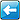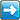This page has been visited 350 times. The contents of this site are (c) their respective owners. If you wish to use anything within, please check their status first. If you have any information, photographs, comments, additions, memorabilia or corrections to this site please contact us